Get Started »

# Differential Equations

The Wolfram Language can find solutions to ordinary, partial and delay differential equations (ODEs, PDEs and DDEs).

DSolveValue takes a differential equation and returns the general solution:

(C stands for a constant of integration.)
 In:=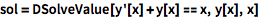⨯ `sol = DSolveValue[y'[x] + y[x] == x, y[x], x]`
 Out=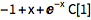Use /. to replace the constant:

 In:=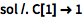⨯ `sol /. C -> 1`
 Out=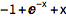Or add conditions for a specific solution:

 In:=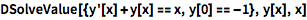⨯ `DSolveValue[{y'[x] + y[x] == x, y == -1}, y[x], x]`
 Out=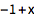NDSolveValue finds numerical solutions:

 In:=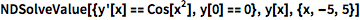⨯ `NDSolveValue[{y'[x] == Cos[x^2], y == 0}, y[x], {x, -5, 5}]`
 Out=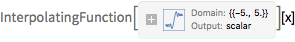You can plot this InterpolatingFunction directly:

 In:=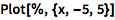⨯ `Plot[%, {x, -5, 5}]`
 Out=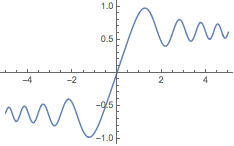To solve systems of differential equations, include all equations and conditions in a list:

(Note that the line breaks have no effect.)
 In:=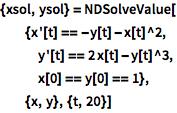⨯ ```{xsol, ysol} = NDSolveValue[ {x'[t] == -y[t] - x[t]^2, y'[t] == 2 x[t] - y[t]^3, x == y == 1}, {x, y}, {t, 20}]```
 Out=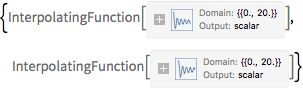Visualize the solution as a parametric plot:

 In:=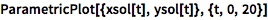⨯ `ParametricPlot[{xsol[t], ysol[t]}, {t, 0, 20}]`
 Out=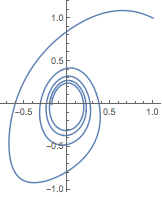QUICK REFERENCE: Differential Equations `»`